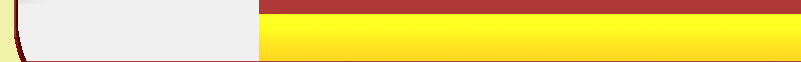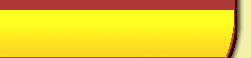# Phrasal Verbs Spanish Vocabulary

### Phrasal Verbs is Verbos Frasales in Spanish## Phrasal Verbs Vocabulary Word Search

Search equivalent of the following english words in Spanish.

, To abide by, To account for, To add up, To advise against, To agree with, To allow for, To appeal to, To apply for, To back away, To back down, To back up, To bank on, To black out, To block off, To blow up, To boil down to, To boot up, To break away, To break down, To break into, To break out, To break out of, To break up, To bring up, To brush up on, To bump into, To burn out, To call back, To call off, To calm down, To carry on, To carry out, To check in, To check out, To clam up, To clamp down on, To come across, To come forward, To count on, To cut down on, To cut out, To deal with, To die down, To do without, To drag on, To draw up, To dress up, To drop in, To drop off, To drop out, To ease off, To end in, To end up, To fall through, To figure out, To fill out, To find out, To focus on, To get along (with), To get at, To get away, To get by, To get in, To get into (+noun), To get off, To get on (well) with (somebody), To get on, To get on with (something), To get out, To get out of, To get over, To get rid of, To get together, To get up, To give in, To give up, To go through, To grow up, To hand in, To hand out, To hang out, To hang up, To hold on, To hurry up, To iron out, To join in, To join up, To keep on, To keep up with, To kick off, To leave out, To let down, To look after, To look down on, To look for, To look forward to, To look on, To look up to, To make fun of, To make up, To mix up, To move in, To move out, To nod off, To own up, To pass away, To pass out, To pay back, To pick up, To point out, To put off, To put on, To put out, To put up, To rely on, To rule out, To run away, To run into, To run out of, To set off, To set up, To shop around, To show off, To show up, To shut up, To sit down, To stand up, To stick up for, To take after, To take care of, To take off, To take on, To take out, To tell off, To think over, To try on, To turn down, To use up, To watch out, To wear out, To wipe off, To work out

 R C O N S U M I R S E E N T R E G A R P I S U P E R A L O J T X C U I D A D O D D K E C H A R S E A T R á S A L í F H F U G A R S E L E H D N A B R E A R E V E E P A G A R C A B E Z A D A S I L L E N A R G L D S E N S V C F P I P L L E G A R A C A T A R C J A I I E C R E E W A N A D K Z N G R L B P C I C E P E A N S R R R I R N E R Y G A Y T R A F I C O T U N E E L T C T I A T N M A B B U S C A R E N A E E L N A E A R D E B R E A T U R R A U J S R L N L Y S R R R A R I R A E L I S S R R N M K N R C á L M A R S E D D R C E D E R R E P S A D V D L D J W G P A T J C O S U G E E S U C E E R T E E O A I T P A P O Y A R E A E D F K E N O U ( R Y E S S N R B L O Q U E A R E C N E N T R A B A N N U A O U M C T A S U L N C Q X U O C O T H T T O A K O N N C N A I I R E J E E O A B A J E N I L A R Z L F M C C Z O I F R A V R N R S E R E D U C E N A U I O C A L M A R S E D R A C R O R E T R O C E D E R L L M N O G O A A E L E I R A E E L E X H U I R S U S A L T I T C E A M L R O J L N G T V P O I O U P C U G A I E R H N R E é D ( C M D A T L A I L I L P Z E I B O R T N A E L N J N B O F N D R á R S O D E J A R R I Z V U T T ) A M T A G I L U H C í A R Z A T M D K R E U R K Y D O O E L N O T S E E M A A A R S G R A C O N T A R C O N A L G U I E N N G S S B R S E P A R A R L L A M A R D E V U E L T A ) I E Y O P E L A F O R Z A R U N A P R O P I E D A D U O G J P Y A L L A N A R C O M E N Z A R D E G O L P E U N E T E S L L E V A R S E B I E N C O N T I N U A R I S H E N T R A R E N ( + S U S T A N T I V O ) X D R E C O G E R V F P W X A H U H G A F W W K U Z I O T L P B U Q D A E J Y Z Q A L C I J Q Y A V Z P X F M R S W N G

More Spanish Vocabulary